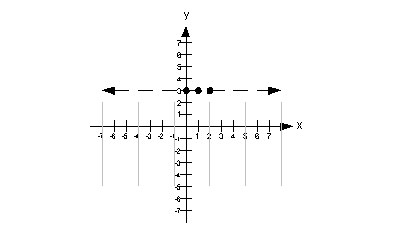# Beginning Algebra Tutorial 24

Beginning Algebra
Tutorial 24: Graphing Linear InequalitiesAnswer/Discussion to 1a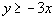Step 1:  Graph the boundary line.

I'm going to use the intercepts to help me graph the boundary line.  Again, you can use any method that you want, unless the directions say otherwise.

When I'm working with only the boundary line, I will put an equal sign between the two sides to emphasize that we are working on the boundary line.  That doesn't mean that I changed the problem. When we put it all together in the end, I will put the inequality back in.

What value is y on the x-intercept?
If you said 0, you are correct.
If you need a review on x-intercepts, go to Tutorial 22: Intercepts.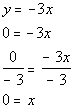*Replace y with 0
*Inverse of mult. by -3 is div. by -3

*x-intercept

x-intercept is (0, 0)

Since the x-intercept came out to be (0, 0), then it stands to reason that when we put in 0 for x to find the y-intercept, we will get (0, 0).

Let's move on and plug in 1 for x to get a second solution: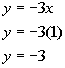*Replace x with 1

(-3, 1) is another solution on the boundary line.

Plug in -1 for x to get a third solution: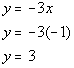*Replace x with -1

(-1, 3) is another solution on the boundary line.

Solutions:

x y (x, y) 0 0 (0, 0) 1 -3 (1, -3) -1 3 (-1, 3)

Since the original problem has a >, this means it DOES include the boundary line.

So are we going to draw a solid or a dashed line for this problem?
It looks like it will have to be a solid line.

Putting it all together, we get the following boundary line for this problem: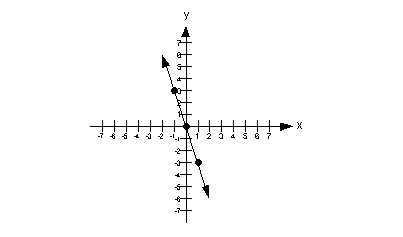Step 2:  Plug in a test point that is not on the boundary line.

Note how the boundary line separates it into two parts.

An easy test point would be (1, 1).  Note that it is a point that is not on the boundary line. In fact, it is located above the boundary line.

Let's put (1, 1) into the original problem and see what happens: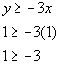*Replacing x and y with 1
*True statement

Since our test point (1, 1) made our inequality TRUE, this means it is a solution.

Our solution would lie above the boundary line.  This means we will shade in the part that is above it.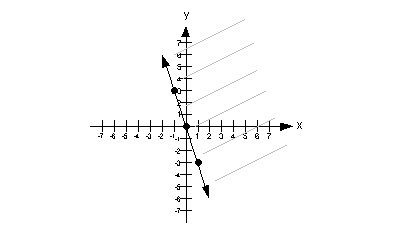Answer/Discussion to 1b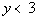Step 1:  Graph the boundary line.

If we wrote this as an equation, it would be y = 3.  This is in the form y = c, which is one of our "special" lines.

Do you remember what type of line y = c graphs as?
It comes out to be a horizontal line.
If you need a review on horizontal lines, go to Tutorial 22: Intercepts

Every y's value on the boundary line would have to be 3.

Solutions:
x y (x, y) 0 3 (0, 3) 1 3 (1, 3) 2 3 (2, 3)

Since the original problem has a <, this means it DOES NOT include the boundary line.

So are we going to draw a solid or a dashed line for this problem?
It looks like it will have to be a dashed line.

Putting it all together, we get the following boundary line for this problem: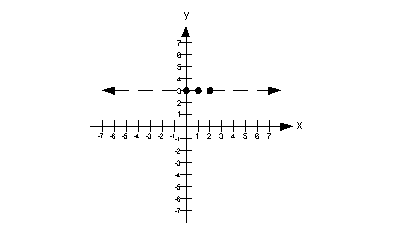Step 2:  Plug in a test point that is not on the boundary line.

Note how the boundary line separates it into two parts.

An easy test point would be (0, 0).  Note that it is a point that is not on the boundary line. In fact, it is located below the boundary line.

Let's put (0, 0) into the original problem and see what happens: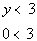*Replace y with 0
*True statement

Since our test point (0, 0) made our inequality TRUE, this means it is a solution.

Our solution would lie below the boundary line.  This means we will shade in the part that is below it